# 线性回归的数学表示

## 监督学习

2104 3 400​
1600​ 3 330​
2400​ 3 369​
1416​ 2 232​
3000​ 4 540​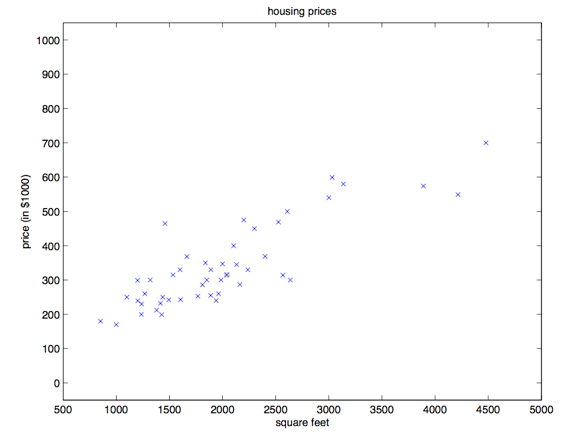价格与房屋面积数据散点图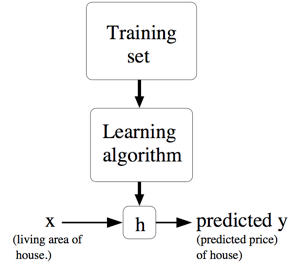监督学习示意图

ISO国际标准推荐使用小写字母加粗或者上标箭头来表示向量。向量一般默认为列向量（Column Vector）。

## 一元线性方程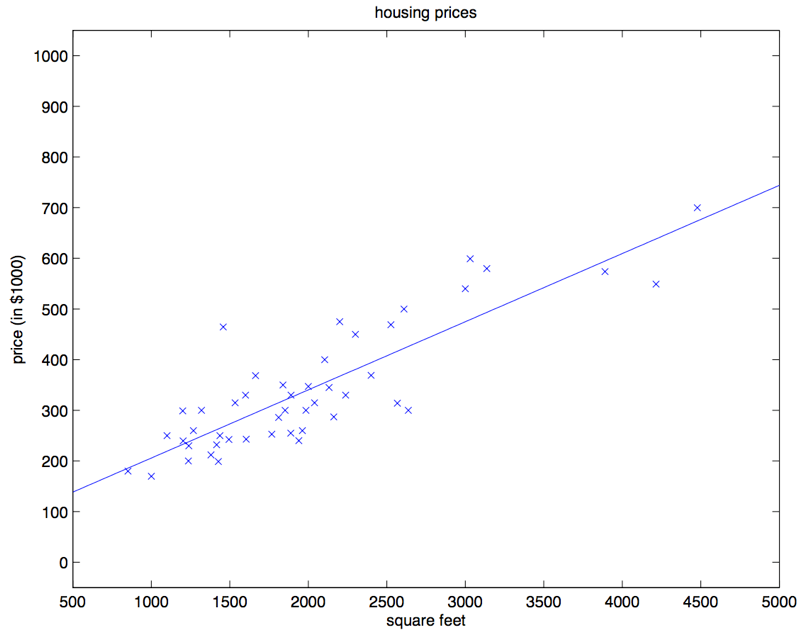$a$$b$可以取不同的参数，到底哪条直线是最佳的呢？如何衡量模型是否以最优的方式拟合数据呢？机器学习用损失函数（Loss Function）的来衡量这个问题。损失函数又称为代价函数（Cost Function），它计算了模型预测值$\hat{y}$和真实值$y$之间的差异程度。从名字也可以看出，这个函数计算的是模型犯错的损失或代价，损失函数越大，模型越差，越不能拟合数据。统计学家通常使用$L(\hat{y}, y)$来表示损失函数。

$L(\hat{y}, y) =\frac{1}{m} \sum_{i=1}^m[(ax^{(i)} + b) - y^{(i)}]^2 \tag{3}$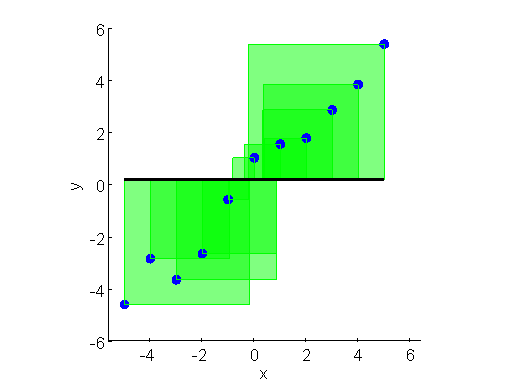假设直线如图，误差的平方和非常大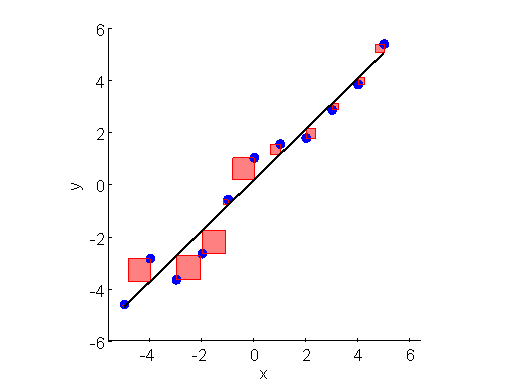假设直线如图，误差的平方和较小

$a^*, b^* = \mathop{\arg\min}_{a, b}L(a, b)$

$a^*, b^* = \mathop{\arg\min}_{a, b}\frac{1}{m}\sum_{i=1}^m[(ax^{(i)} + b) - y^{(i)}]^2 \tag{4}$

• 基于微积分和线性代数知识，求使得$L$导数为0的点，这个点一般为最优点。
• 基于梯度下降，迭代地搜索最优点。

## 线性回归的一般形式

$f_\omega (x) = b + w_1 \times x_1 + w_2 \times x_2 + ... + w_n \times x_n$

$i$条样本有一个需要预测的$\hat{y}^{(i)}$和一组$n$维向量$\boldsymbol{x}^{(i)}$。之前一元回归的参数$m$拓展成了$n$维的向量$\boldsymbol{w}$。这样，某个 $\hat{y}^{(i)}$ 可以表示成$b + \sum_{j=1}^n w_jx_{j}^{(i)}$，其中 $x_{j}^{(i)}$ 表示第 $i$ 个样本的特征向量 $\boldsymbol{x}^{(i)}$ 中第 $j$ 维特征值。 $f(\boldsymbol{x}^{(i)}) = b + \sum_{j=1}^nw_jx_{j}^{(i)}\qquad \qquad b = w_0 \tag{5}$

$f(\boldsymbol{x}^{(i)}) = \sum^n_{j=0} w_j x_{j}^{(i)} = \boldsymbol{w}^T \boldsymbol{x}^{(i)} \tag{6}$

• 直接求解导数为零的点
• 使用梯度下降法